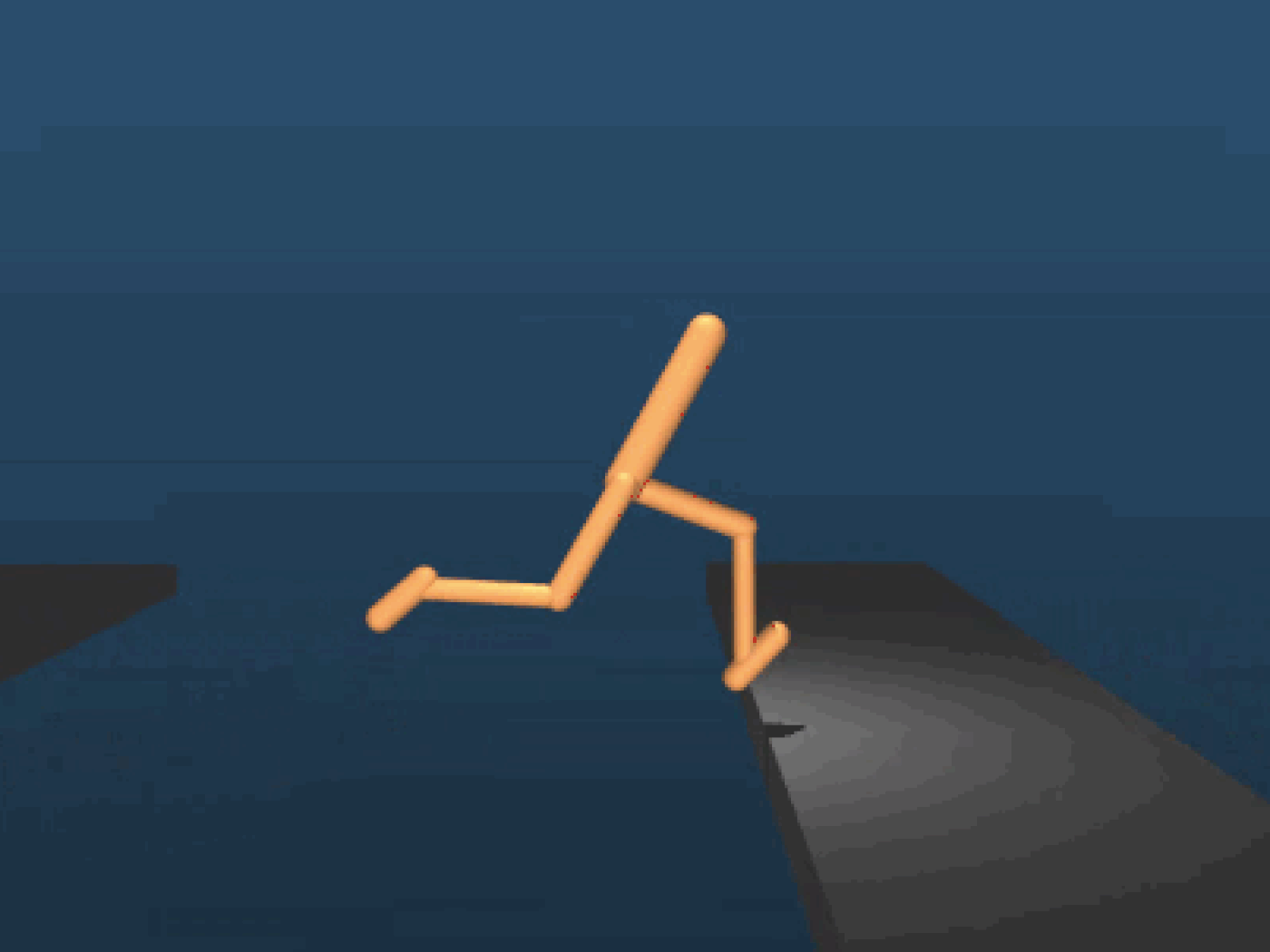# Self Learning AI-Agents Part I: Markov Decision Processes

## A mathematical guide on the theory behind Deep Reinforcement Learning

This is the first article of the multi-part series on self learning AI-Agents or to called it more precisely — Deep Reinforcement Learning. The aim of the series isn’t just to give you an intuition on these topics. Rather I want to provide you with more in depth comprehension of the theory, mathematics and implementation behind the most popular and effective methods of Deep Reinforcement Learning.

#### Self Learning AI-Agents Series — Table of Content

• Part I: Markov Decision Processes (This article)
• Part II: Deep Q-Learning
• Part III: Deep (Double) Q-Learning
• Part IV: Policy Gradients for Continues Action Spaces
• Part V: Dueling Networks
• Part VI: Asynchronous Actor-Critic AgentsFig. 1. AI agent learned how to run and overcome obstacles.

#### Markov Decision Processes — Table of Content

• 0. Introduction
• 1. Reinforcement Learning in a Nutshell
• 2. Markov Decision Processes
• 2.1 Markov Processes
• 2.2 Markov Reward Process
• 2.3 Value Function
• 3. Bellman Equation
• 3.1 Bellman Equation for Markov Reward Processes
• 3.2 Markov Decision Process — Definition
• 3.3 Policies
• 3.4 Action-Value Function
• 3.5 Optimal Policy
• 3.6 Bellman Optimality Equation

### 0. Introduction

Deep reinforcement learning is on the rise. No other sub-field of Deep Learning was more talked about in the recent years – by the researchers as well as the mass media worldwide. Most outstanding achievements in deep learning were made due to deep reinforcement learning. From Google’s Alpha Go that have beaten the worlds best human player in the board game Go (an achievement that was assumed impossible a couple years prior) to DeepMind’s AI agents that teach themselves to walk, run and overcome obstacles (Fig. 1–3).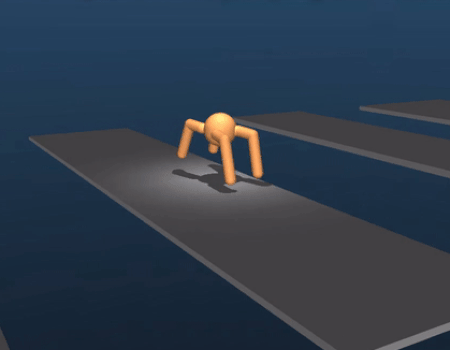Fig. 2. AI agent learned how to run and overcome obstacles.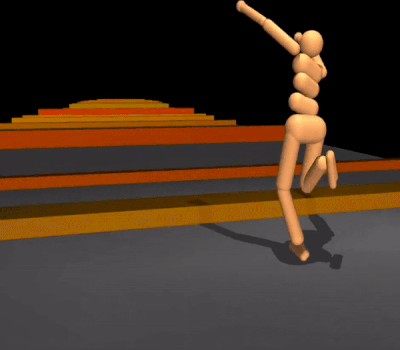Fig. 3. AI agent learned how to run and overcome obstacles.

Other AI agents exceed since 2014 human level performances in playing old school Atari games such as Breakthrough (Fig. 4). The most amazing thing about all of this in my opinion is the fact that none of those AI agents were explicitly programmed or taught by humans how to solve those tasks. They learned it by themselves by the power of deep learning and reinforcement learning. The goal of this first article of the multi-part series is to provide you with necessary mathematical foundation to tackle the most promising areas in this sub-field of AI in the upcoming articles.Fig. 4 AI agent learned how to play Atari’s Breakthrough.

#### 1. Deep Reinforcement Learning in a Nutshell

Deep Reinforcement Learning can be summarized as building an algorithm (or an AI agent) that learns directly from interaction with an environment (Fig. 5). The environment may be the real world, a computer game, a simulation or even a board game, like Go or chess. Like a human the AI Agent learns from consequences of its Actions, rather than from being explicitly taught.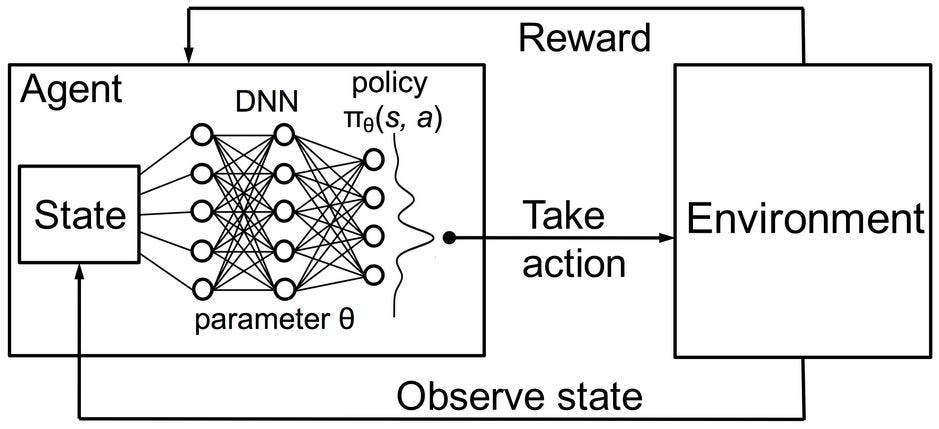Fig. 5 Schematic depiction of deep reinforcement learning

In Deep Reinforcement Learning the Agent is represented by a neural network. The neural network interacts directly with the environment. It observes the current State of the Environment and decides which Action to take (e.g. move left, right etc.) on basis of the current State and the past experiences. Based on the taken Action the AI Agent receives a Reward. The amount of the Reward determines the quality of the taken Action with regards to solving the given problem (e.g. learning how to walk). The objective of an Agent is to learn taking Actions in any given circumstances that maximize the accumulated Reward over time.

### 2. Markov Decision Processes

A Markov Decision Processes (MDP) is a discrete time stochastic control process. MDP is the best approach we have so far to model the complex environment of an AI agent. Every problem that the agent aims to solve can be considered as a sequence of states S1, S2, S3, … Sn (A state may be for example a Go/chess board configuration). The agent takes actions and moves from one state to an other. In the following you will learn the mathematics that determine which action the agent must take in any given situation.

#### 2.1 Markov Processes

A Markov Process is a stochastic model describing a sequence of possible states in which the current state depends on only the previous state. This is also called the Markov Property (Eq. 1). For reinforcement learning it means that the next state of an AI agent only depends on the last state and not all the previous states before.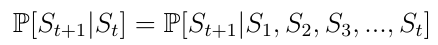Eq. 1 Markov Property

A Markov Process is a stochastic process. It means that the transition from the current state s to the next state s’ can only happen with a certain probability Pss(Eq. 2). In a Markov Process an agent that is told to go left would go left only with a certain probability of e.g. 0.998. With a small probability it is up to the environment to decide where the agent will end up.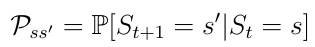Eq. 2 Transition probability from state s to state s’.

Psscan be considered as an entry in a state transition matrix P that defines transition probabilities from all states s to all successor states s’ (Eq. 3).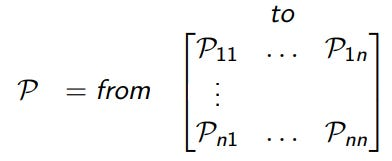Eq. 3. Transition probability matrix.

Remember: A Markov Process (or Markov Chain) is a tuple <S, P> . S is a (finite) set of states. P is a state transition probability matrix.

#### 2.2 Markov Reward Process

A Markov Reward Process is a tuple <S, P, R>. Here R is the reward that the agent expects to receive in the state s (Eq. 4). This process is motivated by the fact that for an AI agent that aims to achieve a certain goal e.g. winning a chess game, certain states (game configurations) are more promising than others in terms of strategy and potential to win the game.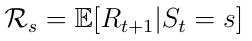Eq. 4. Expected reward in a state s.

The primary topic of interest is the total reward Gt (Eq. 5) which is the expected accumulated reward the agent will receive across the sequence of all states. Every reward is weighted by so called discount factor γ ∈ [0, 1]. It is mathematically convenient to discount rewards since it avoids infinite returns in cyclic Markov processes. Besides the discount factor means the more we are in the future the less important the rewards become, because the future is often uncertain. If the reward is financial, immediate rewards may earn more interest than delayed rewards. Besides animal/human behavior shows preference for immediate reward.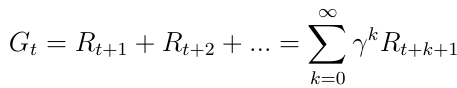Eq. 5. Total reward across all states.

#### 2.3 Value Function

An other important concept is the the one of the value function v(s). The value function maps a value to each state s. The value of a state s is defined as the expected total reward the AI agent will receive if it starts its progress in the state s (Eq. 6).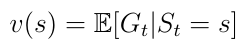Eq. 6. Value function, the expected return starting in state s.

The value function can be decomposed into two parts:

• The immediate reward R(t+1) the agent receives being in state s.
• The discounted value v(s(t+1)) of the next state after the state s.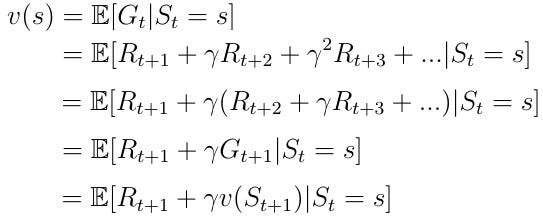Eq. 7 Decomposition of the value function.

### 3. Bellman Equation

#### 3.1 Bellman Equation for Markov Reward Processes

The decomposed value function (Eq. 8) is also called the Bellman Equation for Markov Reward Processes. This function can be visualized in a node graph (Fig. 6). Starting in state s leads to the value v(s). Being in the state s we have certain probability Pss’ to end up in the next state s’. In this particular case we have two possible next states. To obtain the value v(s) we must sum up the values v(s’) of the possible next states weighted by the probabilities Pss’ and add the immediate reward from being in state s. This yields Eq. 9, which is nothing else than Eq.8 if we execute the expectation operator E in the equation.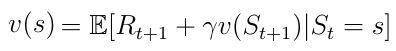Eq. 8 Decomposed value function.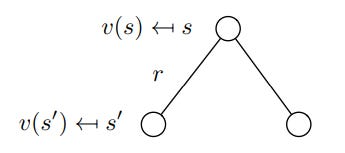Fig. 6 Stochastic transition from s to s’.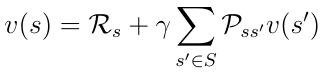Eq. 9 Bellman Equation after execution of the expectation operator E.

#### 3.2 Markov Decision Process — Definition

A Markov Decision Process is a Markov Reward Process with decisions. A Markov Decision Process is described by a set of tuples <S, A, P, R >, A being a finite set of possible actions the agent can take in the state s. Thus the immediate reward from being in state s now also depends on the action a the agent takes in this state (Eq. 10).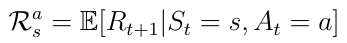Eq. 10 Expected reward depending on the action in a state s.

#### 3.3 Policies

At this point we shall discuss how the agent decides which action must be taken in a particular state. This is determined by the so called policy π (Eq. 11). Mathematically speaking a policy is a distribution over all actions given a state s. The policy determines the mapping from a state s to the action a that must be taken by the agent.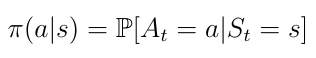Eq. 11 Policy as a mapping from s to a.

Remember: Intuitively speaking the policy π can be described as a strategy of the agent to select certain actions depending on the current state s.

The policy leads to a new definition of the the state-value function v(s) (Eq. 12) which we define now as the expected return starting from state s, and then following a policy π.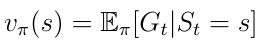Eq. 12 State-value function.

#### 2.7 Action-Value Function

An other important function besides the state-value-function is the so called action-value function q(s,a) (Eq. 13). The action-value function is the expected return we obtain by starting in state s, taking action a and then following a policy π. Notice that for a state s, q(s,a) can take several values since there can be several actions the agent can take in a state s.

Remember: Action-value function tells us how good is it to take a particular action in a particular state.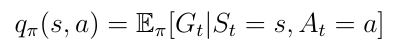Eq. 13 Action-value function.

Previously the state-value function v(s) could be decomposed into the following form: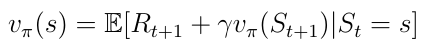Eq. 14 Decomposed state-value function.

The same decomposition can be applied to the action-value function: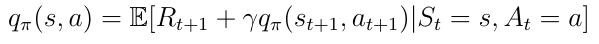Eq. 15 Decomposed action-value function.

At this point lets discuss how v(s) and q(s,a) relate to each other. The relation between these functions can be visualized again in a graph: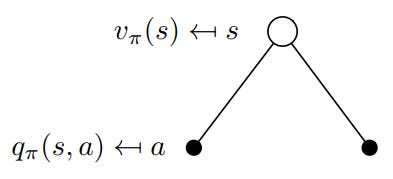Fig. 7 Visualization of the relation between v(s) and q(s,a).

In this example being in the state s allows us to take two possible actions a. By definition taking a particular action in a particular state gives us the action-value q(s,a). The value function v(s) is the sum of possible q(s,a) weighted by the probability (which is non other than the policy π) of taking an action a in the state s (Eq. 16).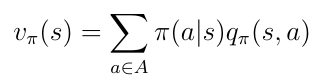Eq. 16 State-value function as weighted sum of action-values.

Now lets consider the opposite case in Fig. 8. The root of the binary tree is now a state in which we choose to take an particular action a. Remember that the Markov Processes are stochastic. Taking an action does not mean that you will end up where you want to be with 100% certainty. Strictly speaking you must consider probabilities to end up in other states after taking the action. In this particular case after taking action a you can end up in two different next states s’: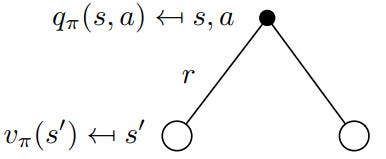Fig. 8 Visualization of the relation between v(s) and q(s,a).

To obtain the action-value you must take the discounted state-values weighted by the probabilities Pss’ to end up in all possible states (in this case only 2) and add the immediate reward: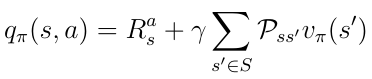Eq. 17 Relation between q(s,a) and v(s).

Now that we know the relation between those function we can insert v(s) from Eq. 16 into q(s,a) from Eq. 17. We obtain Eq. 18 and it can be noticed that there is a recursive relation between the current q(s,a) and next action-value q(s’,a’).Eq. 18 Recursive nature of the action-value function.

This recursive relation can be again visualized in a binary tree (Fig. 9). We begin with q(s,a), end up in the next state s’ with a certain probability Pss’ from there we can take an action a’ with the probability π and we end with the action-value q(s’,a’). To obtain q(s,a) we must go up in the tree and integrate over all probabilities as it can be seen in Eq. 18.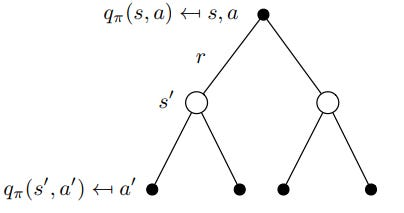Fig. 9 Visualization of the recursive behavior of q(s,a).

#### 2.8 Optimal Policy

The most important topic of interest in deep reinforcement learning is finding the optimal action-value function q*. Finding q* means that the agent knows exactly the quality of an action in any given state. Furthermore the agent can decide upon the quality which action must be taken. Lets define that q* means. The best possible action-value function is the one that follows the policy that maximizes the action-values: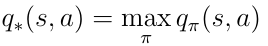Eq. 19 Definition of the best action-value function.

To find the best possible policy we must maximize over q(s, a). Maximization means that we select only the action a from all possible actions for which q(s,a) has the highest value. This yields the following definition for the optimal policy π: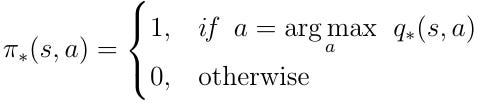Eq. 20 Optimal policy. Take actions that maximize q(s,a).

#### 2.9 Bellman Optimality Equation

The condition for the optimal policy can be inserted into Eq. 18. Thus provides us with the Bellman Optimality Equation: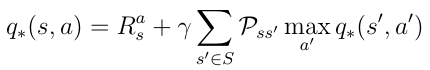Eq. 21 Bellman Optimality Equation

If the AI agent can solve this equation than it basically means that the problem in the given environment is solved. The agent knows in any given state or situation the quality of any possible action with regards to the objective and can behave accordingly.

Solving the Bellman Optimality Equation will be the topic of the upcoming articles. In the following article I will present you the first technique to solve the equation called Deep Q-Learning.

Source: Deep Learning on Medium